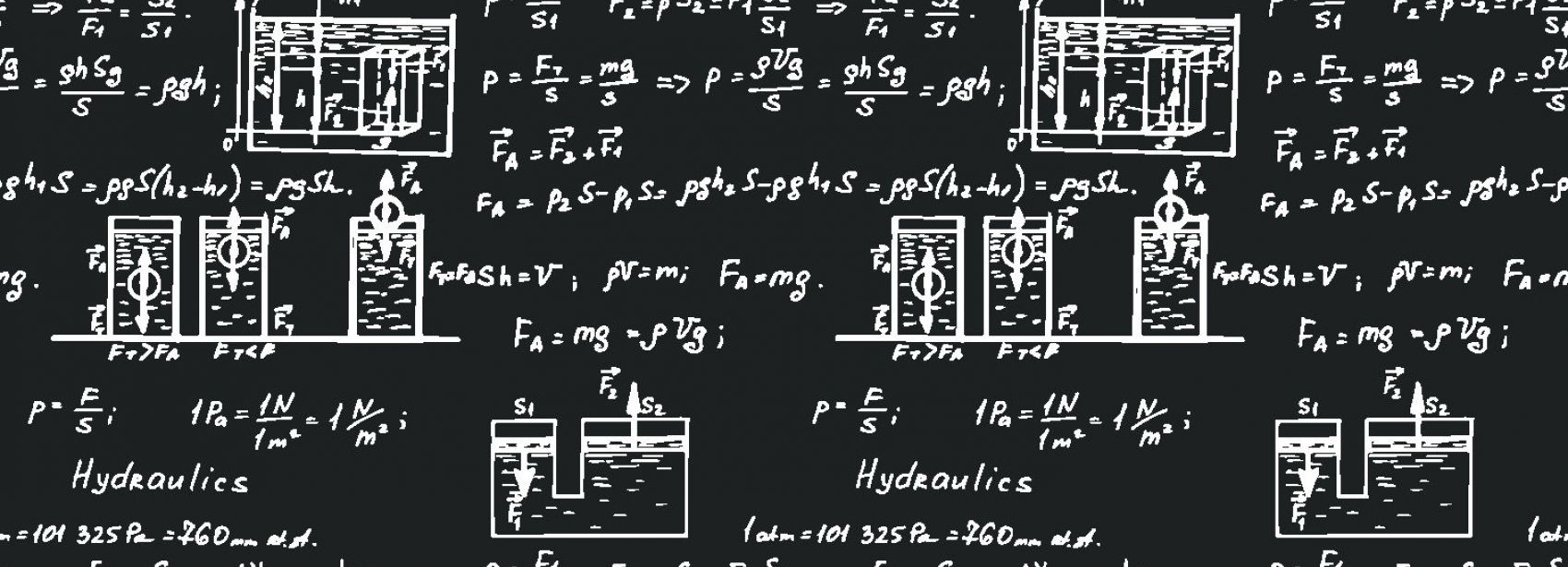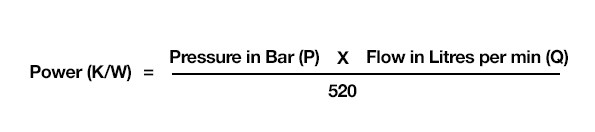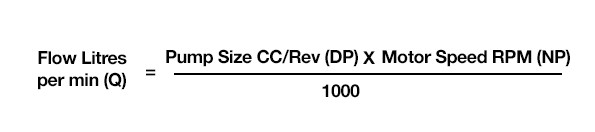# Fluid Power Formulas

#### Fluid power formula’s and basic rules of hydraulics

To start, a few simple principles of hydraulics...

• Pressure is a measure of the resistance to flow

• Load on actuators, flow resistance through pipework and components

• Pressure provides the pushing force

• Transmission of motor torque and cylinder forces are based on PRESSURE

• Oil in a system always takes the path of least resistance

• Actuator movements or leakage is always diverted back to the reservoir

##### Primary mover sizing calculationsTo calculate the size of the primary mover (typically an electric motor or engine), we must first establish the size of the hydraulic pump to give the required flow for the system. Once we have sized the pump, we then know the power required to drive the pump and specify the correct size motor or engine.

When calculating the power input to the pump, the total pump efficiency must be included. This efficiency is the product of volumetric efficiency. The average for axial piston pumps = 0.87. The formula shown (stating a dividing value of 520 allows for an efficiency of 80% on both the pumps and the electric motor) If we were to assume an efficiency of 100% (which it never would be) then the dividing value would be 600.

##### Pump flow calculationsTo calculate the flow capability of a pump in Litres per Min we can use the formula shown.
Remember: 1cm3 = 1ml and 100ml = 1 litre

Note: This is a theoretical value. Actual flow rate will be less due to the volumetric efficiency. Pumps internally leak which is necessary for lubrication, but as internal wear increases the rate of leakage will begin to affect the overall system performance.

• When oil flows from a high pressure to a low pressure without doing work, heat is generated

• Work can be defined as FORCE x DISTANCE MOVED. This process occurs in motors and cylinders through a combination of flow and pressure drop

• For oil to flow in any system, there must always be a pressure difference.

• The pump can only displace oil from a high pressure to a low pressure. All flow processes depend on this rule

• The greater the pressure difference, the greater the flow potential

If you would like some assistance with any of your hydraulic systems design calculations, please call our friendly technical sales team today, +44(0)1172 130042, who are always on hand to offer assistance

Vat Reg Number: 846644204 Company Registration Number: 5230249